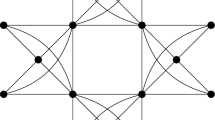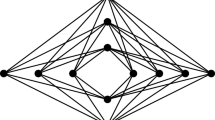# Tight Bounds Towards a Conjecture of Gallai

## Abstract

We prove that for $$n>k\ge 3$$, if G is an n-vertex graph with chromatic number k but any of its proper subgraphs has smaller chromatic number, then G contains at most $$n-k+3$$ copies of a clique of size $$k-1$$. This answers a problem of Abbott and Zhou and provides a tight bound on a conjecture of Gallai.

This is a preview of subscription content, log in via an institution to check access.

Price excludes VAT (USA)
Tax calculation will be finalised during checkout.

### Similar content being viewed by othersYusuf Civan

### The Chromatic Number of a Graph with Two Odd Holes and an Odd Girth

Kaiyang Lan & Feng Liu### New lower bounds on crossing numbers of $$K_{m,n}$$ from semidefinite programming

Daniel Brosch & Sven C. Polak

1. An odd wheel is obtained from an odd cycle C by adding a new vertex x and joining x to every vertex of C. Note that an odd wheel on $$n\ge 6$$ vertices is a 4-critical planar graph and has exactly $$n-1$$ triangles.

2. Note that here we only use $$k-3$$ incidence vectors from A to form $$\textbf{y}_j$$’s. In total, there are $$k-1$$ elements of A that correspond to $$k-1$$ colors. We have two special colors $$k-1$$ and $$k-2$$ set aside after Claim 1 and Claim 2, respectively, which leaves $$k-3$$ colors.

3. If $$e\notin E(G)$$, then it is evident that we have $$t(e,\mathcal {G})=0$$.

## References

1. Abbott, H.L., Zhou, B.: On a conjecture of Gallai concerning complete subgraphs of $$k$$-critical graphs. Discret. Math. 100, 223–228 (1992)

2. Dirac, G.A.: Some theorems on abstract graphs. Proc. Lond. Math. Soc. 2, 69–81 (1952)

3. Jensen, T.R., Toft, B.: Graph Coloring Problems. Wiley, New York (1995)

4. Kézdy, A.E., Snevily, H.S.: On extensions of a conjecture of Gallai. J. Combin. Theory Ser. B 70, 317–324 (1997)

5. Koester, G.: On $$4$$-critical planar graphs with high edge density. Discret. Math. 98, 147–151 (1991)

6. Stiebitz, M.: Subgraphs of colour-critical graphs. Combinatorica 7, 303–312 (1987)

7. Su, X.-Y.: Complete $$(k-1)$$-subgraphs of $$k$$-critical graphs. J. Combin. Combin. Comput. 18, 186–192 (1995)

8. Su, X.-Y.: On complete subgraphs of color-critical graphs. Discret. Math. 143, 243–249 (1995)

## Acknowledgements

The authors would like to thank two referees and Stijn Cambie for their careful reading and for many valuable suggestions.

## Author information

Authors

### Corresponding author

Correspondence to Jie Ma.

### Publisher's Note

Springer Nature remains neutral with regard to jurisdictional claims in published maps and institutional affiliations.

J. Gao: Research supported by the Institute for Basic Science (IBS-R029-C4). J. Ma: Research supported by the National Key R and D Program of China 2020YFA0713100, National Natural Science Foundation of China grant 12125106, Innovation Program for Quantum Science and Technology 2021ZD0302902, and Anhui Initiative in Quantum Information Technologies Grant AHY150200.

## Rights and permissions

Springer Nature or its licensor (e.g. a society or other partner) holds exclusive rights to this article under a publishing agreement with the author(s) or other rightsholder(s); author self-archiving of the accepted manuscript version of this article is solely governed by the terms of such publishing agreement and applicable law.

Reprints and Permissions